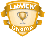# LabVIEW

cancel
Showing results for
Did you mean:

## PID control?

Hi,

I'm doing a project regarding pH stating device. The control program based on LabVIEW 6.1 is roughly done. I also use a simple PID subVI from LabVIEW 6.1 examples to adjust the pH compensation. However, there are a couple issues I still can't figure out.

1. The simple PID subVI needs to know two major values: setpoint and process variable; then it will produce a PID output according to its PID definition:

Output=P*Err + I*Err_Sum + D*dErr/dt.

Now suppose I'm going to compensate a sample's pH from 6.5 back to 7.0. I guess we need a ratio,

Ratio = Current PID Output (numerator)/ Initial PID Output (denominator),

to decide how large acid/base injection rate is needed.

My problem is, what is the "Initial PID Output"? I have been trying many ways to do this. For example, at the first guess, I thought this initial value is the output when we start running the PID controller (setpoint=7/ process variable=6.5), with basic PID parameters which could be p=1, i=0.001, d= 0 or something. Because we only got an output value at the start, this intial value is always "FIXED" during the whole running process. Then let's talk about the ratio's numerator. This numerator is an output value we obtain every dT. Furthermore, we can either use same PID parameters or different PID parameters. Using different PID parameters is to speed up or slow down the process ("p" is the main factor). I'm not quite sure here what this initial output should be used. Do I also need to change i and d when p parameter is changed? Guys, am I correct with this PID ratio idea?

2. pH values I get from a pH meter is in numeral form, i.e. 6.5 and 7.0. In fact, pH is a logarithmic value, i.e 10^-6.5 and 10^-7. Assume I switch to use "logarithmic form" to be our setpoint/process variable and now the process variable comes to "7.1" due to overshoot. According to the above calculation, I will get a very tiny current PID ratio basically because "10^-7.0 - 10^-7.1O"(current error) is quite small compared to "10^-7 - 10^-6.5" (initial error), where a reverse injection is almost nothing effective. The only choice for me is to use numeral form instead of logarithmic form. But the thing is, I can't get optimal p, i, and d parameters because this system is not linear. So, how should I deal with this issue?

Scottie
Message 1 of 3
(2,658 Views)

## Re: PID control?

pH control is not only a mathematical problem. There are also some chemical/physical issues that you should know about.
1/ to make a pH correction, you have to add an acid or alcali solution. the amplitude of the control action should also be a function of the solution concentrations that are used.
2/ the amplitude of the pH variation is of course a function of the amount of acid or alcali added, but also of the buffering properties of the medium. Adding 1 cc of a 1N NaOH solution to 1 liter of water as not the same effect as adding it to 1 liter of 1N sodium acetate solution.
3/ the mixing time should also be taken into account. When acting on a large tank, a fairly large delay between the corrective action and a variation detected by the sensor can affect significantly the efficiency of the control loop
4/ a pH electrode can also have a significant response time, depending on its history.

Of course this is not an answer to your question, but I believe that it will give you a better appreciation of your situation.

CC
Chilly Charly    (aka CC)E-List Master - Kudos glutton - Press the yellow button on the left...

Message 2 of 3
(2,648 Views)

## Re: PID control?

Hello Scottie,

PID control is typically used for linear, time invariant systems, so this might not be the best for your application. You might have some luck using a PID gain scheduler to vary your gains for P I and D as the concentration changes.

Ideally, you could get some model for your system and then mathematically figure out the best gains, but that's not always the easiest to do. I agree that you should first try to get close with mostly proportional gain, and then incorporate the integral and derivative to improve the response.

I wonder if anyone else has already implemented a control systems like this.

Regards,
Vineet A.
Message 3 of 3
(2,603 Views)